# Hensel ring

A commutative local ring to which the Hensel lemma applies, or, according to another definition, to which the implicit function theorem applies. For a local ringwith maximal ideal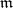the former definition means that for any unitary polynomial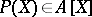and a simple solution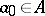of the equation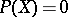() (i.e.and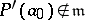) there exists an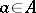such thatand(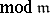).
Examples of Hensel rings include complete local rings, rings of convergent power series (and, in a general sense, analytic rings, cf. Analytic ring), and the ring of algebraic power series (i.e. series fromwhich are algebraic over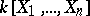). A local ring that is integral over a Hensel ring is a Hensel ring; in particular, a quotient ring of a Hensel ring is a Hensel ring. For any local ringthere exists a general construct — a local Hensel-algebrasuch that for any local Hensel-algebrathere exists a unique homomorphism of-algebras. The algebraof a local ringis a strictly-flat-module,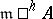will be a maximal ideal of, the residue fields ofandare canonically isomorphic, and the completions ofand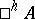(in the topologies of the local rings) coincide. Thus, the ring of algebraic power series inis a Hensel-algebra for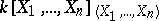. Ifis a Noetherian (or, respectively, reduced, normal, regular, excellent) ring, so is. Conversely, ifis an integral ring,need not be integral; more exactly, there exists a bijective correspondence between the maximal ideals of the integral closure ofand the minimal prime ideals of.
A Hensel ring with a separably-closed residue field is called strictly local (or strictly Henselian), owing to the locality of its spectrum in the étale topology of schemes; in a manner similar to the construction of the Hensel-algebrathere is a strict Hensel-algebra functor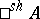. The concept of a Hensel ring may be introduced for a semi-local ring and even, in a more-general sense, for the pair ring–ideal.
A Hensel ring may be described as a ring over which any finite algebra is a direct sum of local rings. Hensel rings were introduced in ; the general theory of Hensel rings and the construction of Hensel-algebras are developed in .
In the theory of étale morphisms and étale topology a Hensel-algebra is understood to be the inductive limit of étale extensions of the ring. In a commutative algebra a Hensel-algebra often replaces the operation of completion, which plays an important role in local studies of objects.